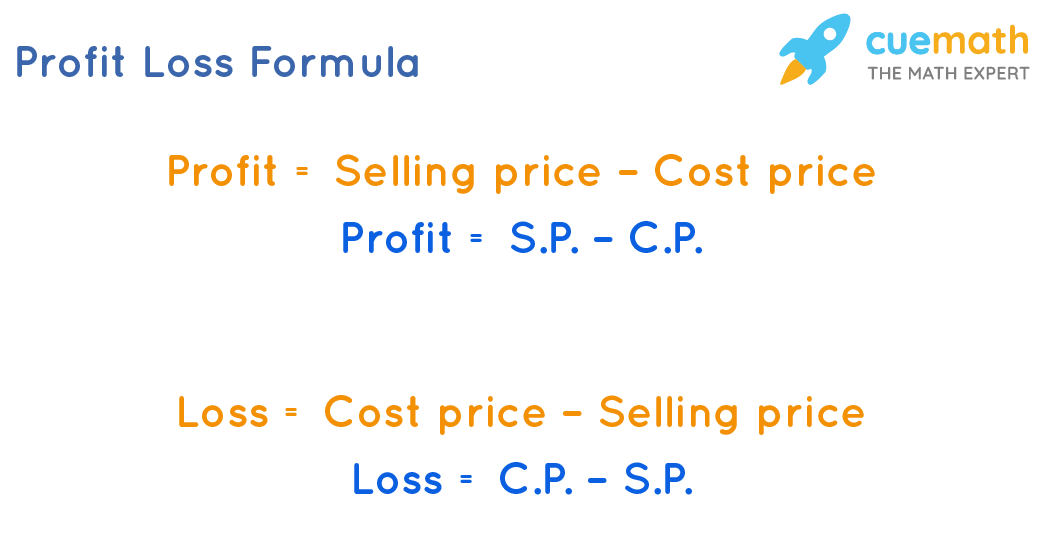# Profit Loss Formula

Go back to  'Math Formulas'

Profit and loss formulas are used to calculate the profit or loss that has been made by selling a particular product. The profit and loss formulas majorly find application in business and financial transactions. Profit and loss as a percentage is generally a measure to depict how much profit or loss a trader incurred from any particular deal.

• When the selling price of any product sold is greater than the cost price (the price at which the product was originally bought), gain or profit is made.
• When the selling price of any product sold is lesser than the cost price (the price at which the product was originally bought), the loss is made.

## What Is the Profit Loss Formula?

Profit Formula for a given cost price and selling price of any article can be expressed as:

Profit = Selling price - Cost price

or,

Profit = S.P. - C.P.

Loss Formula for a given cost price and selling price of any article can be expressed as:

Loss =  Cost price - Selling price

or,

Loss = C.P. - S.P.Some other important formulas related to profit and loss are:

• Profit percentage(P%) = (Profit /Cost Price) × 100
• Loss percentage(L%) = (Loss / Cost price) × 100
• S.P. = {(100 + P%)/100} × CP
• S.P. = {(100 – L%)/100} × CP
• C.P. = {100/(100 + P%)} × SP
• C.P. = {100/(100 – L%)} × SP

## Solved Examples Using Profit Loss Formula

### A shopkeeper buys juice cans in bulk for $30 each. He sells them for$25 each. Calculate the loss and the loss percentage.

Solution:

To find: Loss and Loss Percentage

Given:

Selling price = $30 Cost price =$25

Using Loss Formula,

Loss = $30 -$25

= $5 Using Loss Percentage Formula, Loss Percentage = $$\dfrac{5 \times 100}{25}$$ = 20% Answer: Loss on the given purchase and sale is$5 and loss percentage is 20%.

### On selling a table for $987, Jane loses 6%. For how much did he purchase it? Solution: To find: Cost price of the table Given: Loss Percentage = 6% S.P. =$987

If the loss is 6%, it means that if the cost price is $100, the loss incurred is$6.

S.P. when C.P. is $100 = C.P. - Loss = 100 - 6 =$94

C.P. when S.P. is $94 =$100

CP when SP is $987 = 100/94 × 987 =$1050

Answer:  Cost price of the table = \$1050